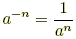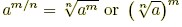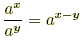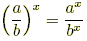# exponent rules

Exponent Rules

Algebra rules and formulas for exponents are listed below.

 Definitions1. an = a·a·a···a  (n times)2. a0 = 1  (a ≠ 0)3.(a ≠ 0)4.(a ≥ 0, m ≥ 0, n > 0) Combining1. multiplication: axay = ax + y2. division:(a ≠ 0)3. powers: (ax)y = axy Distributing   (a ≥ 0, b ≥ 0)1. (ab)x = axbx2.(b ≠ 0) Careful!!1. (a + b)n ≠ an + bn2. (a – b)n ≠ an– bn